• 累计撰写 67 篇文章
• 累计创建 20 个标签
• 累计收到 37 条评论

### 目 录CONTENT2022-05-03 / 6 评论 / 8 点赞 / 1,593 阅读 / 3,652 字

# 一、基本概念

## 1.1 数据流图

• source：源算子，负责数据的读取
• transform：转换算子，负责对数据进行各种的处理加工
• sink：下沉算子，负责数据输出

package tech.kpretty.wordcount;

public class WordCount {
public static void main(String[] args) throws Exception {
// 1.创建执行环境
StreamExecutionEnvironment env = StreamExecutionEnvironment.getExecutionEnvironment();
// 2.处理参数
ParameterTool parameterTool = ParameterTool.fromArgs(args);
// 3.定义数据源
DataStreamSource<String> socketTextStream = env.socketTextStream(parameterTool.get("host"), parameterTool.getInt("port"));
// 4.数据扁平化
SingleOutputStreamOperator<Tuple2<String, Integer>> flatMap = socketTextStream.flatMap((FlatMapFunction<String, Tuple2<String, Integer>>) (s, collector) -> {
String[] words = s.split(",");
for (String word : words) {
collector.collect(Tuple2.of(word, 1));
}
}).returns(Types.TUPLE(Types.STRING, Types.INT));
// 5.分组
KeyedStream<Tuple2<String, Integer>, String> keyBy = flatMap.keyBy(data -> data.f0);
// 6.求和
SingleOutputStreamOperator<Tuple2<String, Integer>> sum = keyBy.sum(1);
// 7.打印
sum.print();
// 8.启动程序
env.execute();
}
}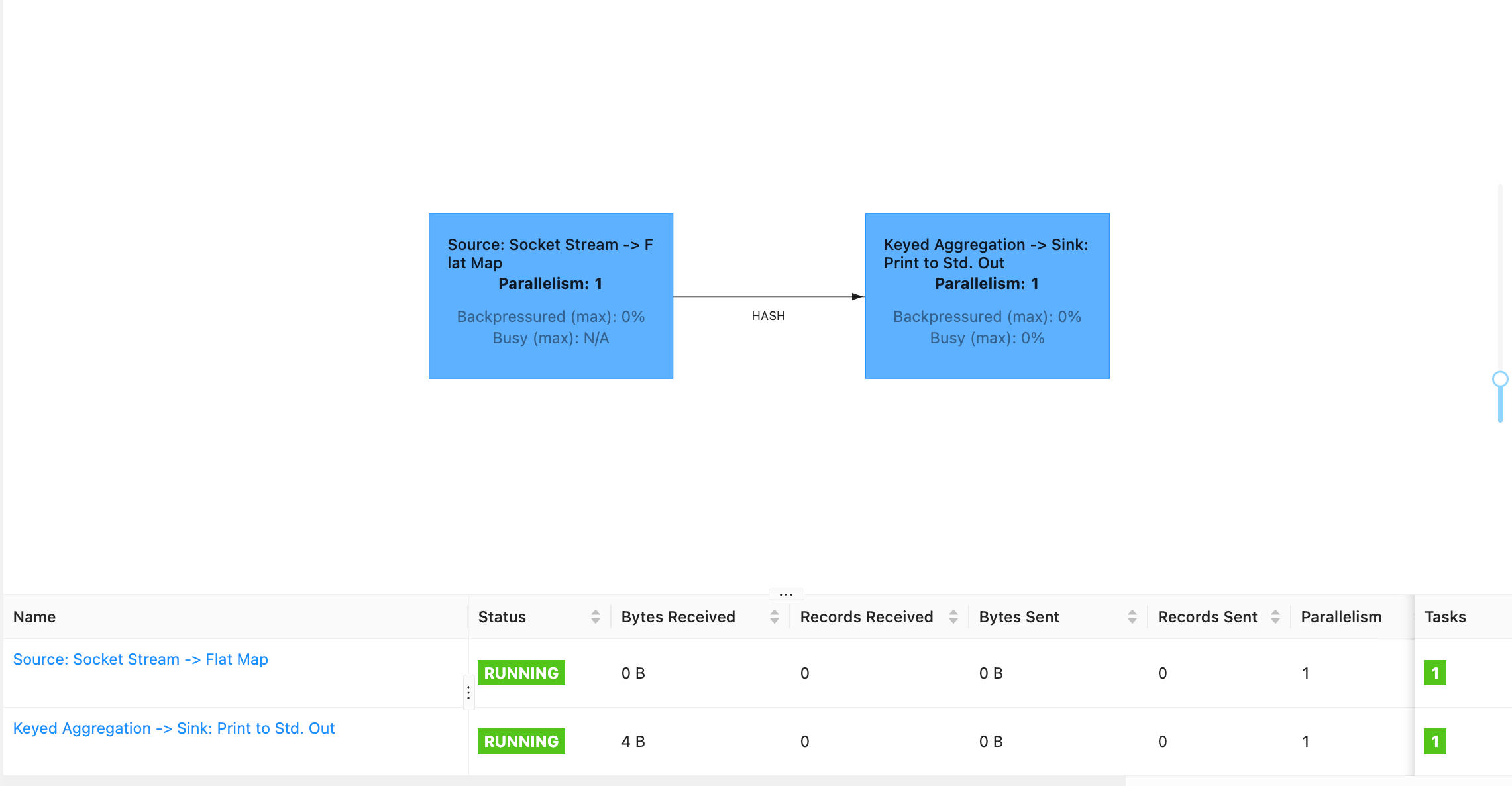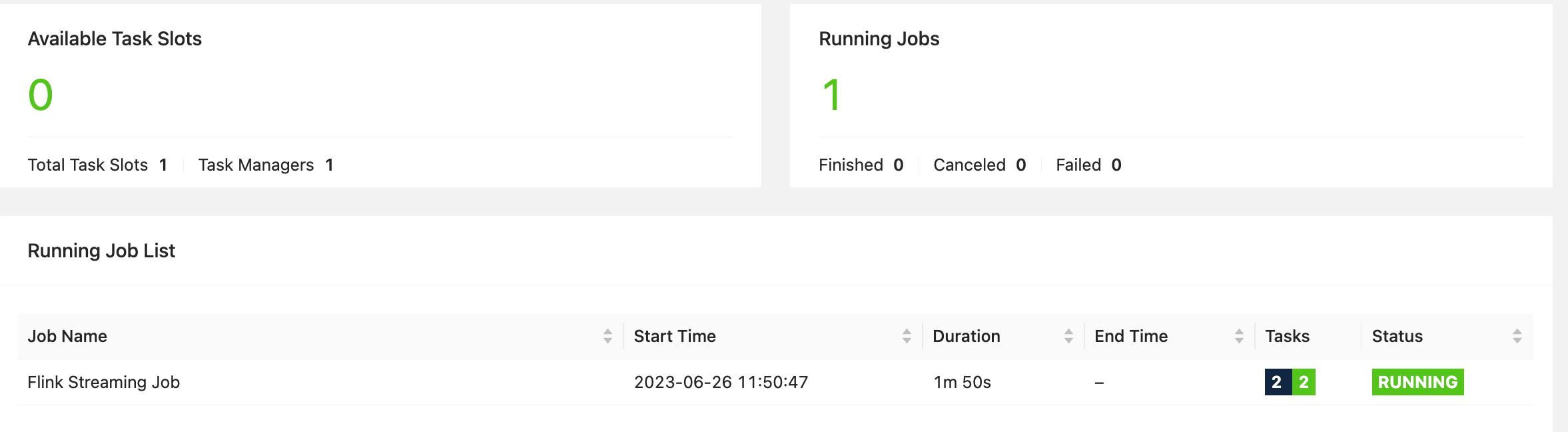## 1.2 并行度和并行子任务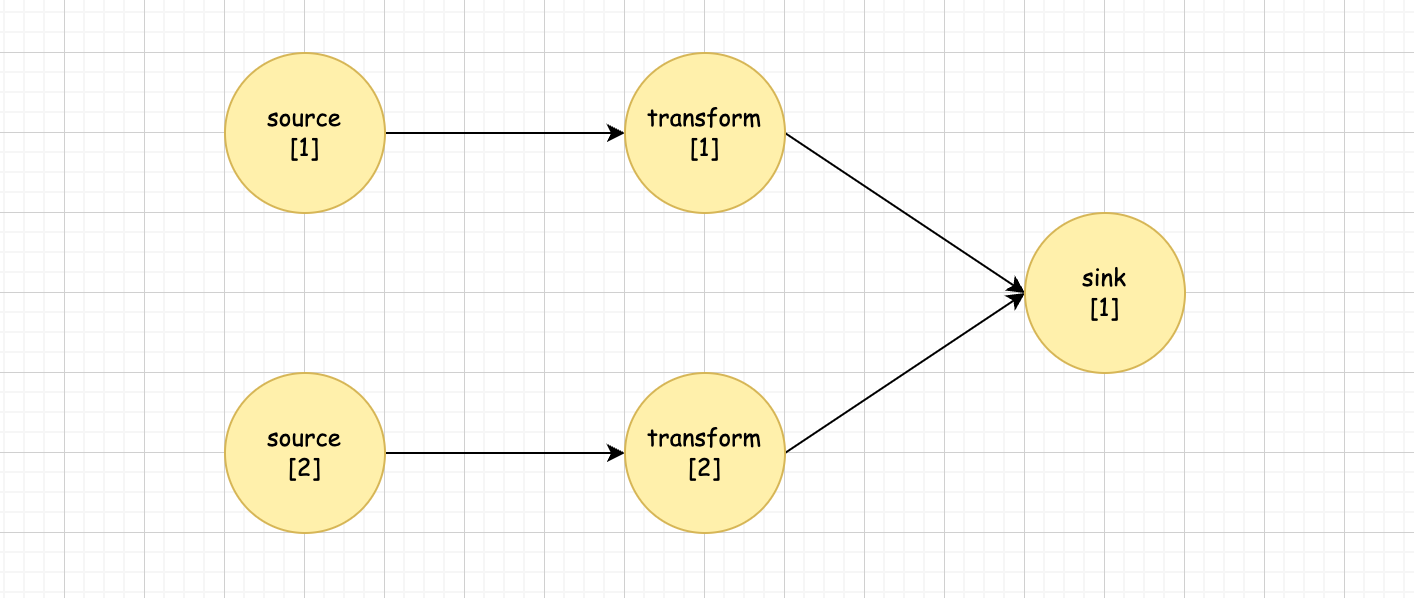## 1.3 算子链

1. 并行度相同
2. one-to-one 操作

# 二、对算子链的魔幻操作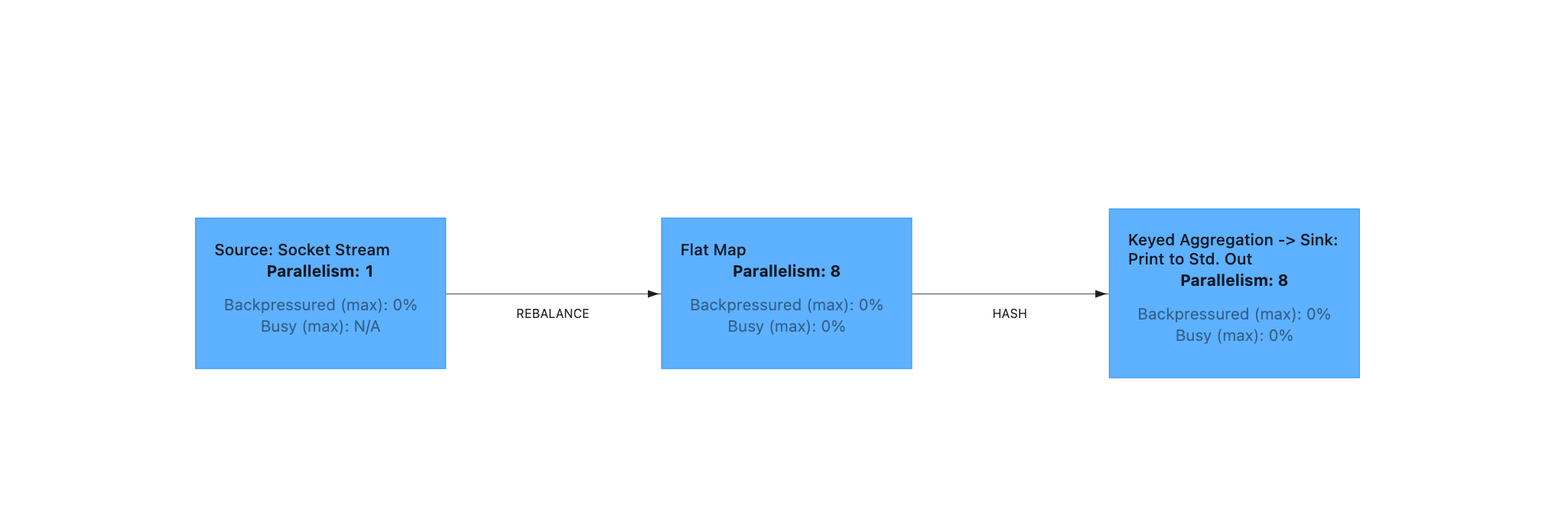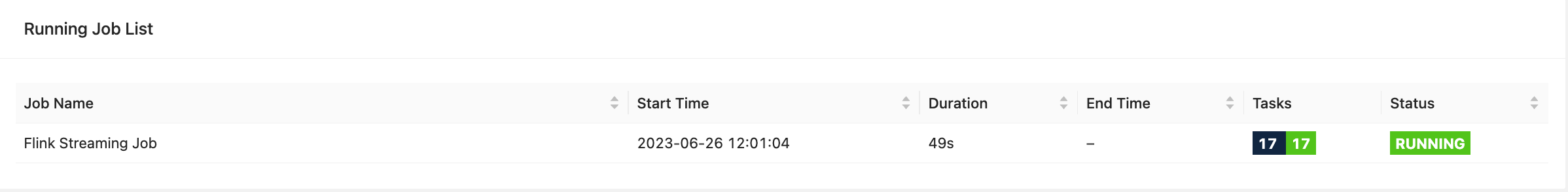package tech.kpretty.wordcount;

public class WordCount {
public static void main(String[] args) throws Exception {
// 1.创建执行环境
StreamExecutionEnvironment env = StreamExecutionEnvironment.getExecutionEnvironment();
// 2.处理参数
ParameterTool parameterTool = ParameterTool.fromArgs(args);
// 3.定义数据源
DataStreamSource<String> socketTextStream = env.socketTextStream(parameterTool.get("host"), parameterTool.getInt("port"));
// 4.数据扁平化
SingleOutputStreamOperator<Tuple2<String, Integer>> flatMap = socketTextStream.flatMap((FlatMapFunction<String, Tuple2<String, Integer>>) (s, collector) -> {
String[] words = s.split(",");
for (String word : words) {
collector.collect(Tuple2.of(word, 1));
}
}).returns(Types.TUPLE(Types.STRING, Types.INT)).disableChaining()
.map((MapFunction<Tuple2<String, Integer>, Tuple2<String, Integer>>) value -> value).returns(Types.TUPLE(Types.STRING, Types.INT))
.map((MapFunction<Tuple2<String, Integer>, Tuple2<String, Integer>>) value -> value).returns(Types.TUPLE(Types.STRING, Types.INT));
// 5.分组
KeyedStream<Tuple2<String, Integer>, String> keyBy = flatMap.keyBy(data -> data.f0);
// 6.求和
SingleOutputStreamOperator<Tuple2<String, Integer>> sum = keyBy.sum(1).startNewChain();
// 7.打印
sum.print();
// 8.启动程序
env.execute();
}
}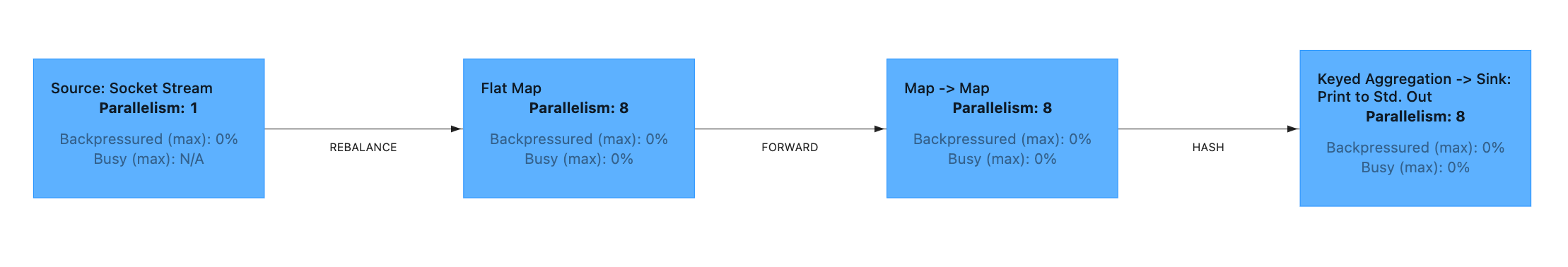Flink 还提供新起一个算子链的方式，例如 flatmap 需要和 source 断开但需要和 map 组成新链，因为 disableChaining 会断开前后

package tech.kpretty.wordcount;

public class WordCount {
public static void main(String[] args) throws Exception {
// 1.创建执行环境
StreamExecutionEnvironment env = StreamExecutionEnvironment.getExecutionEnvironment();
// 2.处理参数
ParameterTool parameterTool = ParameterTool.fromArgs(args);
// 3.定义数据源
DataStreamSource<String> socketTextStream = env.socketTextStream(parameterTool.get("host"), parameterTool.getInt("port"));
// 4.数据扁平化
SingleOutputStreamOperator<Tuple2<String, Integer>> flatMap = socketTextStream.flatMap((FlatMapFunction<String, Tuple2<String, Integer>>) (s, collector) -> {
String[] words = s.split(",");
for (String word : words) {
collector.collect(Tuple2.of(word, 1));
}
}).returns(Types.TUPLE(Types.STRING, Types.INT)).startNewChain()
.map((MapFunction<Tuple2<String, Integer>, Tuple2<String, Integer>>) value -> value).returns(Types.TUPLE(Types.STRING, Types.INT))
.map((MapFunction<Tuple2<String, Integer>, Tuple2<String, Integer>>) value -> value).returns(Types.TUPLE(Types.STRING, Types.INT));
// 5.分组
KeyedStream<Tuple2<String, Integer>, String> keyBy = flatMap.keyBy(data -> data.f0);
// 6.求和
SingleOutputStreamOperator<Tuple2<String, Integer>> sum = keyBy.sum(1).startNewChain();
// 7.打印
sum.print();
// 8.启动程序
env.execute();
}
}


dataflow 如下：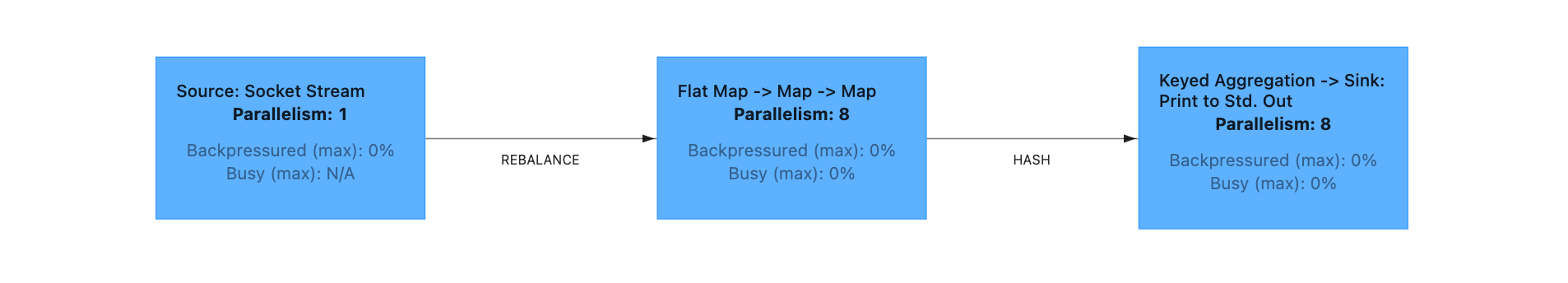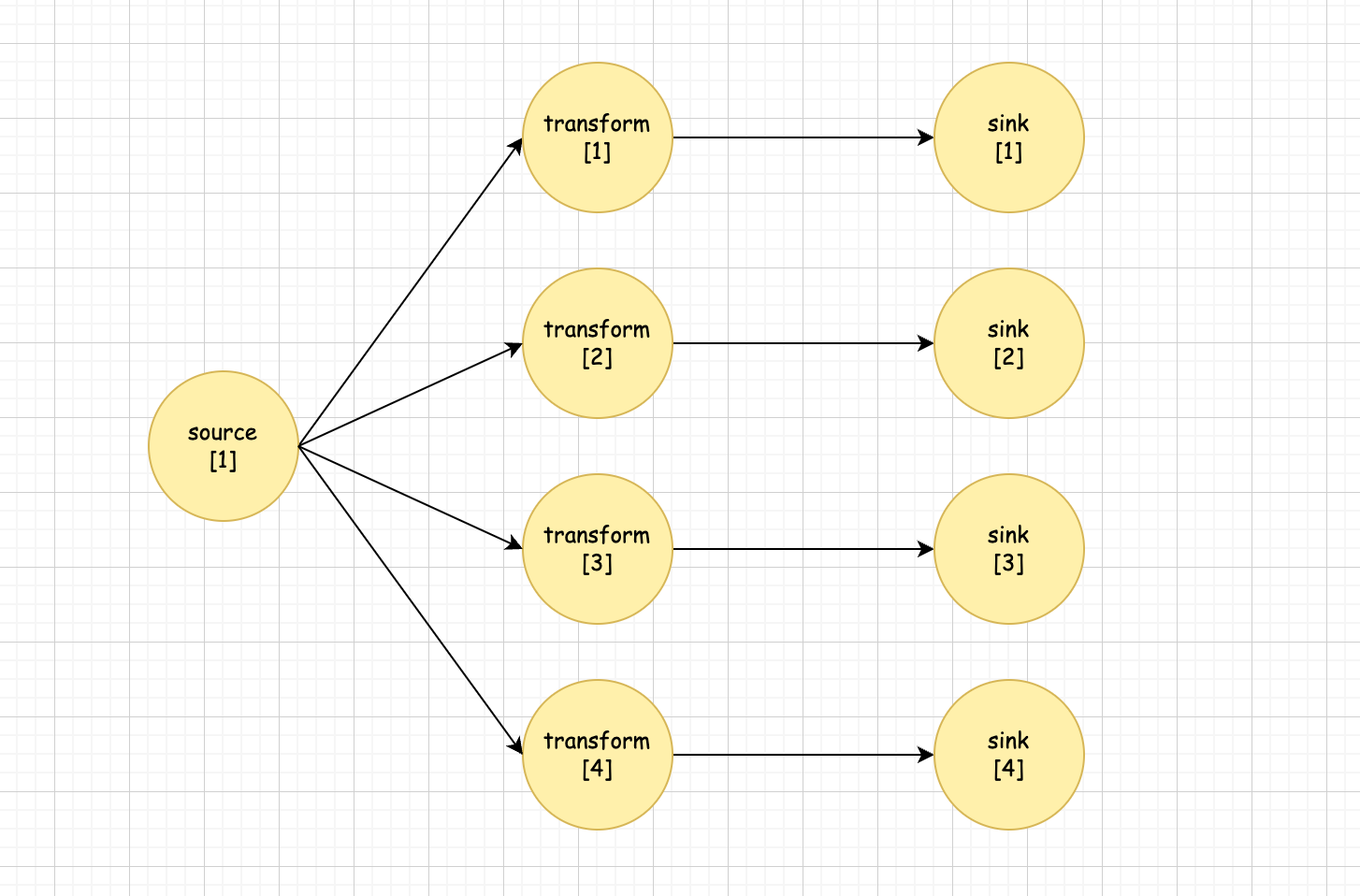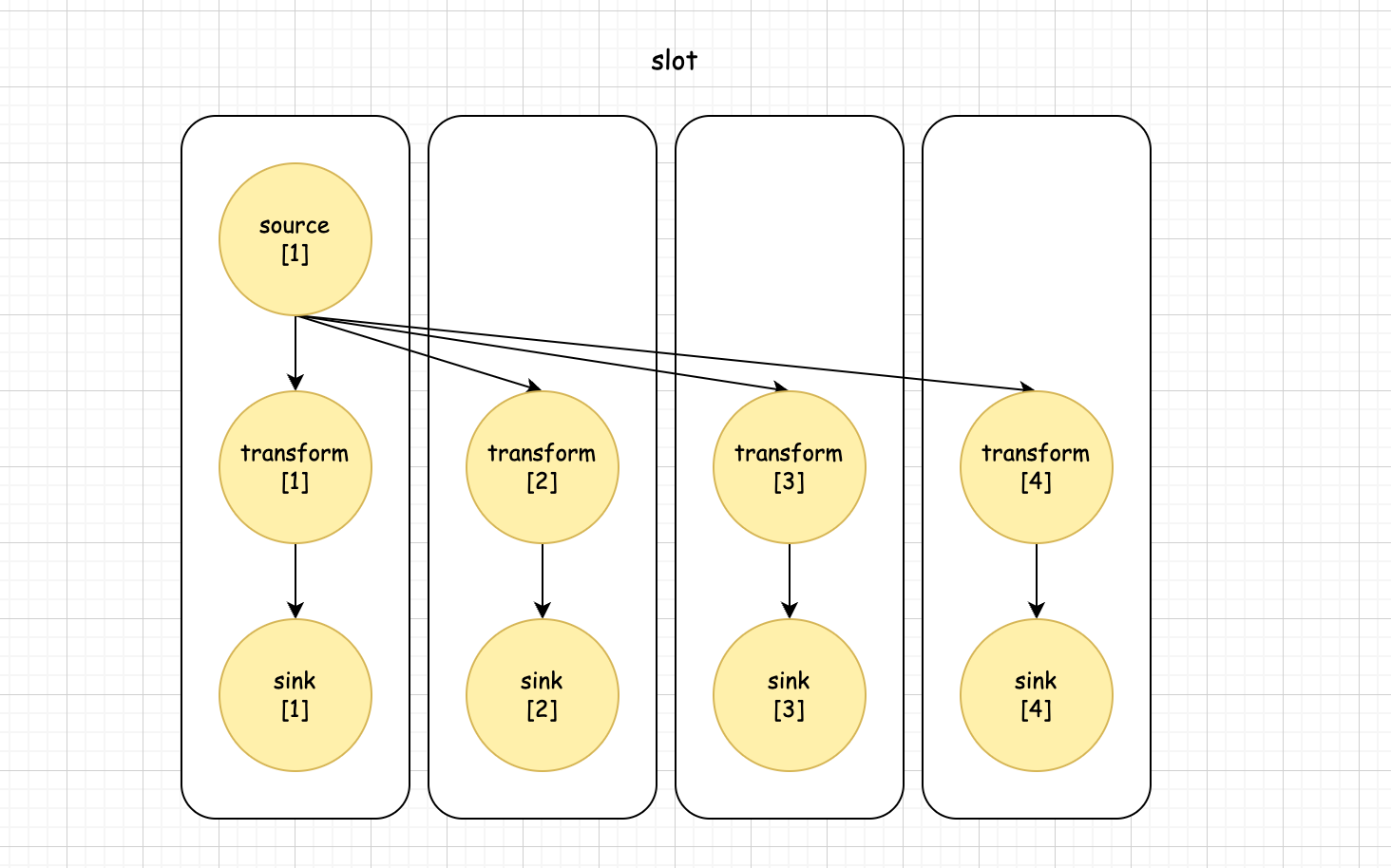package tech.kpretty.wordcount;

public class WordCount {
public static void main(String[] args) throws Exception {
// 1.创建执行环境
StreamExecutionEnvironment env = StreamExecutionEnvironment.getExecutionEnvironment();
// 2.处理参数
ParameterTool parameterTool = ParameterTool.fromArgs(args);
// 3.定义数据源
DataStreamSource<String> socketTextStream = env.socketTextStream(parameterTool.get("host"), parameterTool.getInt("port"));
// 4.数据扁平化
SingleOutputStreamOperator<Tuple2<String, Integer>> flatMap = socketTextStream.flatMap((FlatMapFunction<String, Tuple2<String, Integer>>) (s, collector) -> {
String[] words = s.split(",");
for (String word : words) {
collector.collect(Tuple2.of(word, 1));
}
}).returns(Types.TUPLE(Types.STRING, Types.INT)).slotSharingGroup("slot-group-1");
// 5.分组
KeyedStream<Tuple2<String, Integer>, String> keyBy = flatMap.keyBy(data -> data.f0);
// 6.求和
SingleOutputStreamOperator<Tuple2<String, Integer>> sum = keyBy.sum(1);
// 7.打印
sum.print();
// 8.启动程序
env.execute();
}
}


dataflow如下：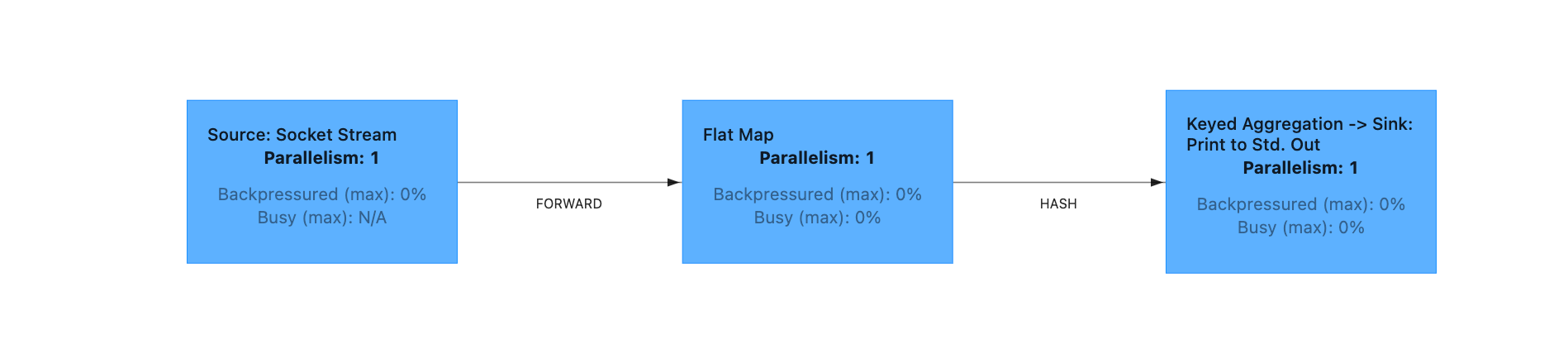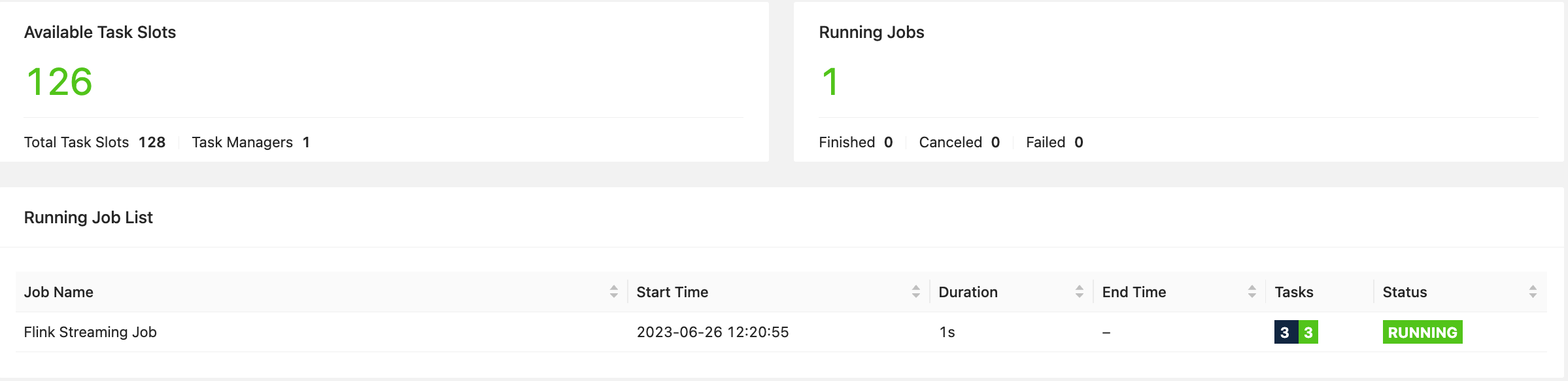8
• 8
• 6